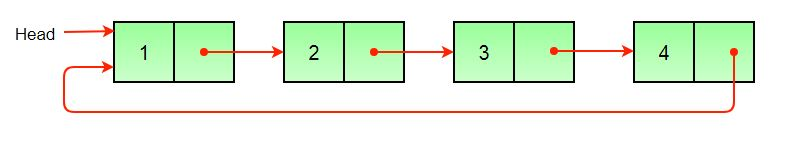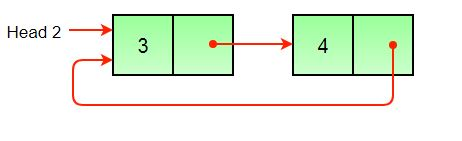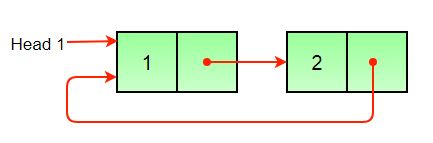GeeksforGeeks App
Open AppBrowser
Continue

# Split a Circular Linked List into two halves`                         Original Linked List  ``                         Result Linked List 1  ``                         Result Linked List 2  `

If there are odd number of nodes, then first list should contain one extra.

Thanks to Geek4u for suggesting the algorithm.
1) Store the mid and last pointers of the circular linked list using tortoise and hare algorithm.
2) Make the second half circular.
3) Make the first half circular.
In the below implementation, if there are odd nodes in the given circular linked list then the first result list has 1 more node than the second result list.

## C++

 `// Program to split a circular linked list``// into two halves``#include ``using` `namespace` `std;` `/* structure for a node */``class` `Node``{``    ``public``:``    ``int` `data;``    ``Node *next;``};` `/* Function to split a list (starting with head)``into two lists. head1_ref and head2_ref are``references to head nodes of the two resultant``linked lists */``void` `splitList(Node *head, Node **head1_ref,``                           ``Node **head2_ref)``{``    ``Node *slow_ptr = head;``    ``Node *fast_ptr = head;``    ` `    ``if``(head == NULL)``        ``return``;``    ` `    ``/* If there are odd nodes in the circular list then``       ``fast_ptr->next becomes head and for even nodes``       ``fast_ptr->next->next becomes head */``    ``while``(fast_ptr->next != head &&``          ``fast_ptr->next->next != head)``    ``{``        ``fast_ptr = fast_ptr->next->next;``        ``slow_ptr = slow_ptr->next;``    ``}``    ` `    ``/* If there are even elements in list``       ``then move fast_ptr */``    ``if``(fast_ptr->next->next == head)``        ``fast_ptr = fast_ptr->next;``        ` `    ``/* Set the head pointer of first half */``    ``*head1_ref = head;``        ` `    ``/* Set the head pointer of second half */``    ``if``(head->next != head)``        ``*head2_ref = slow_ptr->next;``        ` `    ``/* Make second half circular */``    ``fast_ptr->next = slow_ptr->next;``        ` `    ``/* Make first half circular */``    ``slow_ptr->next = head;``}` `/* UTILITY FUNCTIONS */``/* Function to insert a node at ``the beginning of a Circular linked list */``void` `push(Node **head_ref, ``int` `data)``{``    ``Node *ptr1 = ``new` `Node();``    ``Node *temp = *head_ref;``    ``ptr1->data = data;``    ``ptr1->next = *head_ref;``        ` `    ``/* If linked list is not NULL then``       ``set the next of last node */``    ``if``(*head_ref != NULL)``    ``{``        ``while``(temp->next != *head_ref)``        ``temp = temp->next;    ``        ``temp->next = ptr1;``    ``}``    ``else``        ``ptr1->next = ptr1; ``/*For the first node */``    ` `    ``*head_ref = ptr1;``}` `/* Function to print nodes in``a given Circular linked list */``void` `printList(Node *head)``{``    ``Node *temp = head;``    ``if``(head != NULL)``    ``{``        ``cout << endl;``        ``do` `{``        ``cout << temp->data << ``" "``;``        ``temp = temp->next;``        ``} ``while``(temp != head);``    ``}``}` `// Driver Code``int` `main()``{``    ``int` `list_size, i;``        ` `    ``/* Initialize lists as empty */``    ``Node *head = NULL;``    ``Node *head1 = NULL;``    ``Node *head2 = NULL;``    ` `    ``/* Created linked list will be 12->56->2->11 */``    ``push(&head, 12);``    ``push(&head, 56);``    ``push(&head, 2);``    ``push(&head, 11);``    ` `    ``cout << ``"Original Circular Linked List"``;``    ``printList(head);    ``    ` `    ``/* Split the list */``    ``splitList(head, &head1, &head2);``    ` `    ``cout << ``"\nFirst Circular Linked List"``;``    ``printList(head1);``    ` `    ``cout << ``"\nSecond Circular Linked List"``;``    ``printList(head2);``    ``return` `0;``}` `// This code is contributed by rathbhupendra`

## C

 `/* Program to split a circular linked list into two halves */``#include``#include` `/* structure for a node */``struct` `Node``{``  ``int` `data;``  ``struct` `Node *next;``};` `/* Function to split a list (starting with head) into two lists.``   ``head1_ref and head2_ref are references to head nodes of``    ``the two resultant linked lists */``void` `splitList(``struct` `Node *head, ``struct` `Node **head1_ref,``                                            ``struct` `Node **head2_ref)``{``  ``struct` `Node *slow_ptr = head;``  ``struct` `Node *fast_ptr = head;` `  ``if``(head == NULL)``    ``return``;`` ` `  ``/* If there are odd nodes in the circular list then``     ``fast_ptr->next becomes head and for even nodes``     ``fast_ptr->next->next becomes head */``  ``while``(fast_ptr->next != head &&``         ``fast_ptr->next->next != head)``  ``{``     ``fast_ptr = fast_ptr->next->next;``     ``slow_ptr = slow_ptr->next;``  ``} ` ` ``/* If there are even elements in list then move fast_ptr */``  ``if``(fast_ptr->next->next == head)``    ``fast_ptr = fast_ptr->next;     ``  ` `  ``/* Set the head pointer of first half */``  ``*head1_ref = head;   ``     ` `  ``/* Set the head pointer of second half */``  ``if``(head->next != head)``    ``*head2_ref = slow_ptr->next;``  ` `  ``/* Make second half circular */`  `  ``fast_ptr->next = slow_ptr->next;``  ` `  ``/* Make first half circular */`  `  ``slow_ptr->next = head;      ``}` `/* UTILITY FUNCTIONS */``/* Function to insert a node at the beginning of a Circular``   ``linked list */``void` `push(``struct` `Node **head_ref, ``int` `data)``{``  ``struct` `Node *ptr1 = (``struct` `Node *)``malloc``(``sizeof``(``struct` `Node));``  ``struct` `Node *temp = *head_ref;``  ``ptr1->data = data; ``  ``ptr1->next = *head_ref;``  ` `  ``/* If linked list is not NULL then set the next of``    ``last node */``  ``if``(*head_ref != NULL)``  ``{``    ``while``(temp->next != *head_ref)``      ``temp = temp->next;       ``    ``temp->next = ptr1;``  ``}``  ``else``     ``ptr1->next = ptr1; ``/*For the first node */` `  ``*head_ref = ptr1;    ``}` `/* Function to print nodes in a given Circular linked list */``void` `printList(``struct` `Node *head)``{``  ``struct` `Node *temp = head;``  ``if``(head != NULL)``  ``{``    ``printf``(``"\n"``);``    ``do` `{``      ``printf``(``"%d "``, temp->data);``      ``temp = temp->next;``    ``} ``while``(temp != head);``  ``}``}` `/* Driver program to test above functions */``int` `main()``{``  ``int` `list_size, i;``  ` `  ``/* Initialize lists as empty */``  ``struct` `Node *head = NULL;``  ``struct` `Node *head1 = NULL;``  ``struct` `Node *head2 = NULL; ` `  ``/* Created linked list will be 12->56->2->11 */``  ``push(&head, 12);``  ``push(&head, 56);  ``  ``push(&head, 2);  ``  ``push(&head, 11);  ` `  ``printf``(``"Original Circular Linked List"``);``  ``printList(head);     `` ` `  ``/* Split the list */``  ``splitList(head, &head1, &head2);`` ` `  ``printf``(``"\nFirst Circular Linked List"``);``  ``printList(head1); ` `  ``printf``(``"\nSecond Circular Linked List"``);``  ``printList(head2); ``  ` `  ``getchar``();``  ``return` `0;``}`

## Java

 `// Java program to delete a node from doubly linked list``import` `java.util.*;``import` `java.io.*;` `public` `class` `LinkedList {` `    ``static` `Node head, head1, head2;` `    ``static` `class` `Node {` `        ``int` `data;``        ``Node next, prev;` `        ``Node(``int` `d)``        ``{``            ``data = d;``            ``next = prev = ``null``;``        ``}``    ``}` `    ``/* Function to split a list (starting with head) into``     ``two lists. head1_ref and head2_ref are references to``     ``head nodes of the two resultant linked lists */``    ``void` `splitList()``    ``{``        ``Node slow_ptr = head;``        ``Node fast_ptr = head;` `        ``if` `(head == ``null``) {``            ``return``;``        ``}` `        ``/* If there are odd nodes in the circular list then``         ``fast_ptr->next becomes head and for even nodes``         ``fast_ptr->next->next becomes head */``        ``while` `(fast_ptr.next != head``               ``&& fast_ptr.next.next != head) {``            ``fast_ptr = fast_ptr.next.next;``            ``slow_ptr = slow_ptr.next;``        ``}` `        ``/* If there are even elements in list then move``         ``* fast_ptr */``        ``if` `(fast_ptr.next.next == head) {``            ``fast_ptr = fast_ptr.next;``        ``}` `        ``/* Set the head pointer of first half */``        ``head1 = head;` `        ``/* Set the head pointer of second half */``        ``if` `(head.next != head) {``            ``head2 = slow_ptr.next;``        ``}``        ``/* Make second half circular */``        ``fast_ptr.next = slow_ptr.next;` `        ``/* Make first half circular */``        ``slow_ptr.next = head;``    ``}` `    ``/* Function to print nodes in a given singly linked list``     ``*/``    ``void` `printList(Node node)``    ``{``        ``Node temp = node;``        ``if` `(node != ``null``) {``            ``do` `{``                ``System.out.print(temp.data + ``" "``);``                ``temp = temp.next;``            ``} ``while` `(temp != node);``        ``}``    ``}` `    ``public` `static` `void` `main(String[] args)``    ``{``        ``LinkedList list = ``new` `LinkedList();` `        ``// Created linked list will be 12->56->2->11``        ``list.head = ``new` `Node(``12``);``        ``list.head.next = ``new` `Node(``56``);``        ``list.head.next.next = ``new` `Node(``2``);``        ``list.head.next.next.next = ``new` `Node(``11``);``        ``list.head.next.next.next.next = list.head;` `        ``System.out.println(``            ``"Original Circular Linked list "``);``        ``list.printList(head);` `        ``// Split the list``        ``list.splitList();``        ``System.out.println(``""``);``        ``System.out.println(``"First Circular List "``);``        ``list.printList(head1);``        ``System.out.println(``""``);``        ``System.out.println(``"Second Circular List "``);``        ``list.printList(head2);``    ``}``}` `// This code has been contributed by Mayank Jaiswal`

## Python3

 `# Python program to split circular linked list into two halves` `# A node structure``class` `Node:``    ` `    ``# Constructor to create a new node``    ``def` `__init__(``self``, data):``        ``self``.data ``=` `data``        ``self``.``next` `=` `None`  `# Class to create a new  Circular Linked list``class` `CircularLinkedList:``    ` `    ``# Constructor to create a empty circular linked list``    ``def` `__init__(``self``):``        ``self``.head ``=` `None` `    ``# Function to insert a node at the beginning of a``    ``# circular linked list``    ``def` `push(``self``, data):``        ``ptr1 ``=` `Node(data)``        ``temp ``=` `self``.head``        ` `        ``ptr1.``next` `=` `self``.head` `        ``# If linked list is not None then set the next of``        ``# last node``        ``if` `self``.head ``is` `not` `None``:``            ``while``(temp.``next` `!``=` `self``.head):``                ``temp ``=` `temp.``next``            ``temp.``next` `=` `ptr1` `        ``else``:``            ``ptr1.``next` `=` `ptr1 ``# For the first node` `        ``self``.head ``=` `ptr1` `    ``# Function to print nodes in a given circular linked list``    ``def` `printList(``self``):``        ``temp ``=` `self``.head``        ``if` `self``.head ``is` `not` `None``:``            ``while``(``True``):``                ``print` `(``"%d"` `%``(temp.data),end``=``' '``)``                ``temp ``=` `temp.``next``                ``if` `(temp ``=``=` `self``.head):``                    ``break`  `    ``# Function to split a list (starting with head) into``    ``# two lists. head1 and head2 are the head nodes of the``    ``# two resultant linked lists``    ``def` `splitList(``self``, head1, head2):``        ``slow_ptr ``=` `self``.head``        ``fast_ptr ``=` `self``.head``    ` `        ``if` `self``.head ``is` `None``:``            ``return``        ` `        ``# If there are odd nodes in the circular list then``        ``# fast_ptr->next becomes head and for even nodes``        ``# fast_ptr->next->next becomes head``        ``while``(fast_ptr.``next` `!``=` `self``.head ``and``            ``fast_ptr.``next``.``next` `!``=` `self``.head ):``            ``fast_ptr ``=` `fast_ptr.``next``.``next``            ``slow_ptr ``=` `slow_ptr.``next` `        ``# If there are even elements in list then``        ``# move fast_ptr``        ``if` `fast_ptr.``next``.``next` `=``=` `self``.head:``            ``fast_ptr ``=` `fast_ptr.``next` `        ``# Set the head pointer of first half``        ``head1.head ``=` `self``.head` `        ``# Set the head pointer of second half``        ``if` `self``.head.``next` `!``=` `self``.head:``            ``head2.head ``=` `slow_ptr.``next` `        ``# Make second half circular``        ``fast_ptr.``next` `=` `slow_ptr.``next``    ` `        ``# Make first half circular``        ``slow_ptr.``next` `=` `self``.head`  `# Driver program to test above functions` `# Initialize lists as empty``head ``=` `CircularLinkedList()``head1 ``=` `CircularLinkedList()``head2 ``=` `CircularLinkedList()` `head.push(``12``)``head.push(``56``)``head.push(``2``)``head.push(``11``)` `print` `(``"Original Circular Linked List"``)``head.printList()` `# Split the list``head.splitList(head1 , head2)` `print` `(``"\nFirst Circular Linked List"``)``head1.printList()` `print` `(``"\nSecond Circular Linked List"``)``head2.printList()` `# This code is contributed by Nikhil Kumar Singh(nickzuck_007)`

## C#

 `// C# program to delete a node``// from doubly linked list``using` `System;``class` `GFG``{``    ``public` `Node head, head1, head2;` `    ``public` `class` `Node``    ``{``        ``public` `int` `data;``        ``public` `Node next, prev;` `        ``public` `Node(``int` `d)``        ``{``            ``data = d;``            ``next = prev = ``null``;``        ``}``    ``}` `    ``/* Function to split a list (starting with head)``    ``into two lists. head1_ref and head2_ref are``    ``references to head nodes of the two``    ``resultant linked lists */``    ``void` `splitList()``    ``{``        ``Node slow_ptr = head;``        ``Node fast_ptr = head;` `        ``if` `(head == ``null``)``        ``{``            ``return``;``        ``}` `        ``/* If there are odd nodes in the``        ``circular list then fast_ptr->next``        ``becomes head and for even nodes``        ``fast_ptr->next->next becomes head */``        ``while` `(fast_ptr.next != head &&``               ``fast_ptr.next.next != head)``        ``{``            ``fast_ptr = fast_ptr.next.next;``            ``slow_ptr = slow_ptr.next;``        ``}` `        ``/* If there are even elements in list``           ``then move fast_ptr */``        ``if` `(fast_ptr.next.next == head)``        ``{``            ``fast_ptr = fast_ptr.next;``        ``}` `        ``/* Set the head pointer of first half */``        ``head1 = head;` `        ``/* Set the head pointer of second half */``        ``if` `(head.next != head)``        ``{``            ``head2 = slow_ptr.next;``        ``}``        ` `        ``/* Make second half circular */``        ``fast_ptr.next = slow_ptr.next;` `        ``/* Make first half circular */``        ``slow_ptr.next = head;``    ``}` `    ``/* Function to print nodes``    ``in a given singly linked list */``    ``void` `printList(Node node)``    ``{``        ``Node temp = node;``        ``if` `(node != ``null``)``        ``{``            ``do``            ``{``                ``Console.Write(temp.data + ``" "``);``                ``temp = temp.next;``            ``} ``while` `(temp != node);``        ``}``    ``}` `    ``public` `static` `void` `Main(String[] args)``    ``{``        ``GFG list = ``new` `GFG();` `        ``// Created linked list will be 12->56->2->11``        ``list.head = ``new` `Node(12);``        ``list.head.next = ``new` `Node(56);``        ``list.head.next.next = ``new` `Node(2);``        ``list.head.next.next.next = ``new` `Node(11);``        ``list.head.next.next.next.next = list.head;` `        ``Console.WriteLine(``"Original Circular Linked list "``);``        ``list.printList(list.head);` `        ``// Split the list``        ``list.splitList();``        ``Console.WriteLine(``""``);``        ``Console.WriteLine(``"First Circular List "``);``        ``list.printList(list.head1);``        ``Console.WriteLine(``""``);``        ``Console.WriteLine(``"Second Circular List "``);``        ``list.printList(list.head2);``    ``}``}` `// This code is contributed by PrinciRaj1992`

## Javascript

 ``

Output:

```Original Circular Linked List
11 2 56 12